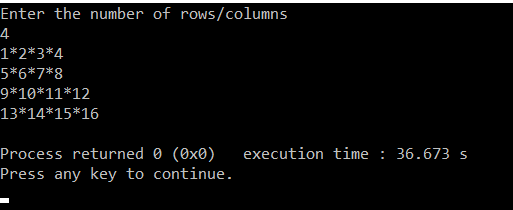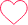# Print Number Star Square Pattern Type1

1*2*3*4

5*6*7*8

9*10*11*12

13*14*15*16

## PREREQUISITE:

Basic knowledge of C language and use of loops.

## ALGORITHM:

1. Take the number of rows/columns as input from the user and store it in any variable.(‘l‘ in this case).
2. Run a loop ‘l’ number of times to iterate through each of the rows. From i=0 to i<l. The loop should be structured as for( i=0 ; i<l : i++).
3. Inside this loop run another nested loop to iterate through the columns. From j=0 to j<l. The loop should be structured as for(j=0 ; j<l ; j++).
4. increment count and the run an if condition if(j==l-1) .
5. which means if it is the last column then print only count
6. Else print a star after count
7. outside the loop print a newline

## CODE IN C:

```#include<stdio.h>
int main()
{
int i,j,l,count=0;                             //declaring integers i,j for loops and l for number of rows
printf("Enter the number of rows/columns\n");  //Asking user for input
scanf("%d",&l);                                //Taking the input for number of rows
for(int i=0;i<l;i++)                           //Outer loop for number of rows
{
for(int j=0;j<l;j++)                       //Inner loop for number of columns in each row
{
count++;                                //incrementing count
if(j==l-1)                              //running if statement to not print star after the last column of digits
{
printf("%d",count);                //printing count
}
else                                   //else statement to print star after count
{
printf("%d*",count);               //printing star after count
}
}
printf("\n");                               //Printing a new line after each row has been printed.
}
}```

## DISPLAYING OUTPUT:### One comment on “Print Number Star Square Pattern Type1”

•Mohit

Python:
m=1
for i in range(4):
for j in range(4):
print(‘%d*’%m,end=”)
m+=1
print()0